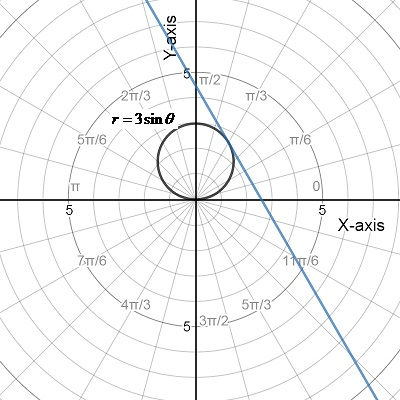Chapter 10.4, Problem 67E

Chapter
Section
Textbook Problem

Finding Slopes of Tangent Lines Using Technology In Exercises 65-68, use a graphing utility to (a) graph the polar equation, (b) draw the tangent line at the given value of θ , and (c) find dy/dx at the given value of θ. (Hint: Let the increment between the values of θ equal π / 2 4 .) r = 3 sin θ , θ = π 3

(a)

To determine

The graph of the given polar equation with the help of the graphing utility

Explanation

Given: The polar equation is r=3sinθ and the angle is θ=π3

(b)

To determine

Sketch the tangent value at given value of θ.(c)

To determine

The value dydx at given value of θ.

Still sussing out bartleby?

Check out a sample textbook solution.

See a sample solution

The Solution to Your Study Problems

Bartleby provides explanations to thousands of textbook problems written by our experts, many with advanced degrees!

Get Started

Solve the equations in Exercises 112 for x (mentally, if possible). x1=cx+d(c1)

Finite Mathematics and Applied Calculus (MindTap Course List)

Find the median for the following set of scores: 1, 9, 3, 6, 4, 3, 11, 10

Essentials of Statistics for The Behavioral Sciences (MindTap Course List)

Rationalize the numerator: x1x1.

Applied Calculus for the Managerial, Life, and Social Sciences: A Brief Approach

Factor each expression in Problems 7-18 as a product of binomials. 15.

Mathematical Applications for the Management, Life, and Social Sciences

Evaluate the limit, if it exists. limh01(xh)21x2h

Single Variable Calculus: Early Transcendentals